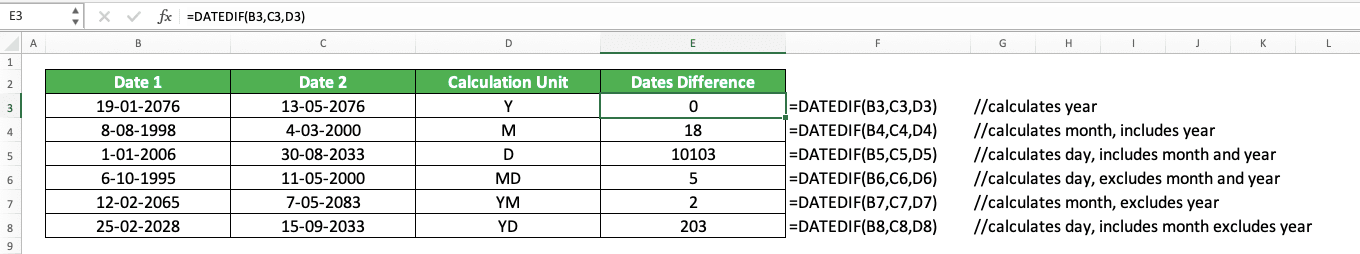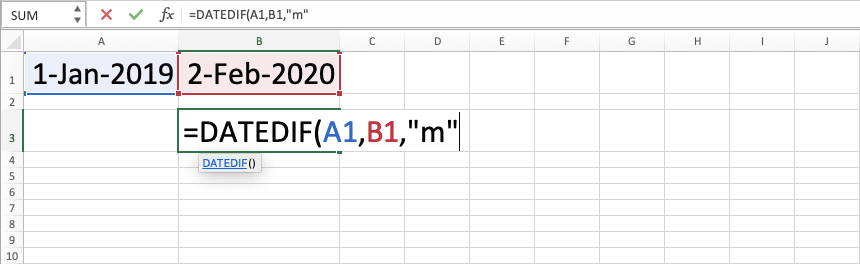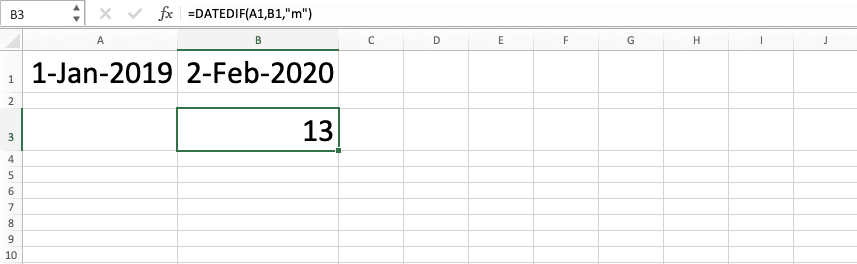How to Use the DATEDIF Formula in Excel: Functions, Examples and Writing Steps - Compute Expert

# How to Use the DATEDIF Formula in Excel: Functions, Examples and Writing Steps

Home >> Excel Tutorials from Compute Expert >> Excel Formulas List >> How to Use the DATEDIF Formula in Excel: Functions, Examples and Writing Steps

In this tutorial, you will learn how to use the DATEDIF formula in excel completely.

When you work with dates in excel, you may sometimes want to get the difference between your two dates. You might need that difference in a day, month, or year unit depending on your calculation purpose.

If you want to use an excel formula to make your dates difference calculation much easier, then you can use DATEDIF. However, you should understand how to use it first as it can be easy to get your DATEDIF formula writing wrong.

Want to master the way to use DATEDIF so you can utilize it to help your dates calculation in excel? Read this tutorial until its last part!

Disclaimer: This post may contain affiliate links from which we earn commission from qualifying purchases/actions at no additional cost for you. Learn more

## What is the DATEDIF Formula in Excel?

DATEDIF is an excel formula you can use to calculate the difference between two dates. You can say DATEDIF is a “hidden” formula in excel since you won’t get suggestions from excel when you type it.

## DATEDIF Function in Excel

You can use DATEDIF to get the difference between two dates in a day, month, or year unit. You can specify the way you want to do the dates difference calculation process too with this DATEDIF formula.

## DATEDIF Result

The DATEDIF result is a number that represents the difference between the two dates you input into it.

## Excel Version from Which We Can Start Using DATEDIF

You can use DATEDIF in excel since excel 2003.

## The Way to Write It and Its Inputs

Here is the general writing form of DATEDIF in excel.

= DATEDIF ( earlier_date , later_date , calculation_unit_code )

And here is a bit explanation of the inputs we need to give to DATEDIF.
• earlier_date = the date which is earlier between the two dates you want to get the difference of
• later_date = the date which is later between the two dates you want to get the difference of
• calculation_unit_code = the unit code which will determine whether you get a day, month, or year unit from the calculation. It will also determine the way DATEDIF will calculate your dates difference

## Unit Code Input Options in DATEDIF

Here is a summary of the calculation unit code input options you can give to DATEDIF and their meaning. Make sure you input the right unit code so you will get the result you want from your DATEDIF!

Yyear-
Myearincludes year
Ddayincludes month and year
MDdayexcludes month and year
YMmonthexcludes year
YDdayexcludes year

What do the words “include” and “exclude” mean in the additional note column? They mean DATEDIF will include or exclude the unit numbers we mention there in its calculation process to get your result. It will translate the unit numbers it includes to the unit you want for your result before it does its calculation.

To understand the concept easier, see the following example. Let’s say you calculate the difference of 1 January 2011 and 2 February 2012 with DATEDIF. Here are the results you will get from each calculation unit code.
• Y = 1 (calculate the difference of your dates years (2012-2011))
• M = 13 (calculate the difference of your dates months by considering their years too (2-1 + (2012-2011)x12)
• D = 397 (calculate the difference of your dates years by considering their years and months too (2-1 + 31 (the days’ difference between February and January) + (2012-2011)x365))
• MD = 1 (calculate the difference of your dates days (2-1))
• YM = 1 (calculate the difference of your dates months (2-1))
• YD = 32 (calculate the difference of your dates days by considering their months too (2-1 + 31 (the days’ difference between February and January))

## Example of Its Usage and Result

Here is a usage and result example of DATEDIF in excel.As you can see, we can get our date difference by using DATEDIF in the example. The result we get depends on the calculation unit code we input to the DATEDIF.

## Writing Steps

Want to get some guidance when you write your DATEDIF formula in excel? See its writing steps below!

1. Type an equal sign ( = ) in the cell where you want to put your DATEDIF result2. Type DATEDIF (can be with large and small letters) and an open bracket sign after =3. Input the earlier date between the two dates you want to get the difference of. Then, type a comma sign ( , )4. Input the later date between the two dates you want to get the difference of. Then, type a comma sign ( , )5. Input the calculation unit code you want. See the code options you can input with their meaning in the previous section of this tutorial6. Type a close bracket sign7. Press Enter
8. Done!## DATEDIF Not Working? Possible Reasons and Solutions

Do you get the wrong result from your DATEDIF? Do you get an error? Here are the things that can be the reason and their solution.

• Reason: You input your earlier and later date in DATEDIF in a wrong order
Solution: Check whether you input your later date before your earlier date in DATEDIF. If that is the case, reverse the order of your date inputs

• Reason: You input the wrong or invalid calculation unit code in your DATEDIF
Solution: Check again the calculation unit code you can input in DATEDIF in the previous section of this tutorial. Have you input one of them and have you inputted the right one to get the calculation result you want? If not, then change your input with the appropriate calculation unit code

• Reason: Excel doesn’t recognize your DATEDIF date inputs as dates
Solution: If you input your dates directly to DATEDIF, use the excel DATE formula to be safer. If you input your dates through cell coordinates, check again and try to retype the dates writing in your cells

Check again your DATEDIF formula writing for these points and make sure you have written it correctly!

## Calculate Age from Date of Birth Using DATEDIF: DATEDIF TODAY

Want to get the current age of a person from his/her date of birth in excel? You can do that easily by combining DATEDIF and TODAY in your formula writing.

Here is the general writing form of the formulas’ combination to calculate age from date of birth.

= DATEDIF ( date_of_birth , TODAY() , calculation_unit_code )

TODAY is a formula that helps you to get an updated today’s date. By combining it with DATEDIF, you will get an age number that keeps updating to today’s date!

You should input the calculation unit code that gets you the type of age you want in DATEDIF. Usually, your calculation unit code input will be Y to get the years difference between today and the date of birth. You can input M or D too if you want the month or day age instead.

You can also get a complete age with the year, month, and day unit with DATEDIF and TODAY. If you want this, then you can write the formula below to get what you want.

= DATEDIF ( date_of_birth , TODAY() , “Y” ) & “ years ” & DATEDIF ( date_of_birth , TODAY() , “YM” ) & “ months ” & DATEDIF ( date_of_birth , TODAY() , “MD” ) & “ days”

The “Y” calculation unit in DATEDIF will give you the age years. Meanwhile, “YM” will give you the age months and “MD” will give you the age days. By concatenating them with the “years”, “months”, and “days” text also, you will get a clear and complete age result!

Here is the formula writing implementation example in excel.As you can see, we can get our age calculation result from the date of birth by combining DATEDIF and TODAY. Just write the two formulas’ combination in the pattern we have discussed before!

## Exercise

After you have learned how to use DATEDIF in excel completely, you can practice your understanding by doing this exercise!

### Questions

Use DATEDIF to answer all the questions below.
1. What is the difference between the years in the second row?
2. What is the difference between the months by calculating the years too in the third row?
3. What is the difference between the days by ignoring the months and years in the fourth row?

DATEDIF makes it easy to get dates difference in excel in the unit that you want. If you subtract your dates manually, you will just get the days difference.

On the other hand, if you just want your dates’ days difference, you may want to just subtract them. It might be simpler for you that way!

Related tutorials you should learn too:

Get updated excel info from Compute Expert by registering your email. It's free!